# Direct Current Circuit Analysis – Gibilisco MCQs in Electronics

(Last Updated On: December 8, 2017)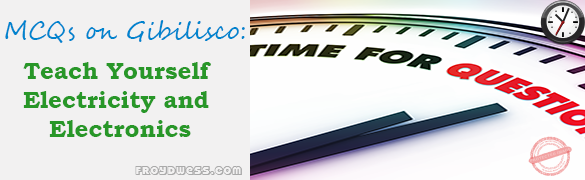This is the Multiple Choice Questions (MCQs) in Chapter 5: Direct Current Circuit Analysis from the book Teach Yourself Electricity and Electronics, 5th edition by Stan Gibilisco. If you are looking for a reviewer in Electronics Engineering this will definitely help you before taking the Board Exam.

### Start Practice Exam Test Questions

1. In a series-connected string of holiday ornament bulbs, if one bulb gets shorted out, which of these is most likely?

• A. All the other bulbs will go out.
• B. The current in the string will go up.
• C. The current in the string will go down.
• D. The current in the string will stay the same.

NOTE: For questions 2, 3, 8, 9, see Fig. 5-9.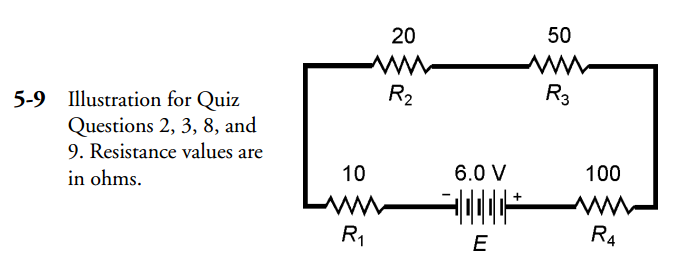2. Four resistors are connected in series across a 6.0-V battery. The values are R1 = 10 Ω, R2 = 20 Ω, R3 = 50 Ω, and R4 = 100 Ω as shown in Fig. 5-9. The voltage across R2 is:

• A. 0.18 V.
• B. 33 mV.
• C. 5.6 mV.
• D. 670 mV.

3. In question 2 (Fig. 5-9), the voltage across the combination of R3 and R4 is:

• A. 0.22 V.
• B. 0.22 mV.
• C. 5.0 V.
• D. 3.3 V.

NOTE: For questions 4, 5, 6, 7, 10 and 11 see Fig. 5-10.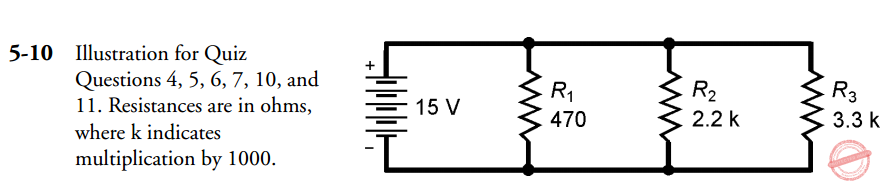4. Three resistors are connected in parallel across a battery that delivers 15 V. The values are R1 = 470 Ω, R2 = 2.2 KΩ, R3 = 3.3 KΩ(Fig. 5-10). The voltage across R2 is:

• A. 4.4 V.
• B 5.0 V.
• C. 15 V.
• D. Not determinable from the data given.

5. In the example of question 4 (Fig. 5-10), what is the current through R2?

• A. 6.8 mA.
• B. 43 mA.
• C. 150 mA.
• D. 6.8 A.

6. In the example of question 4 (Fig. 5-10), what is the total current drawn from the source?

• A. 6.8 mA.
• B. 43 mA.
• C. 150 mA.
• D. 6.8 A.

7. In the example of question 4 (Fig. 5-10), suppose that resistor R2 opens up. The current through the other two resistors will:

• A. Increase.
• B. Decrease.
• C. Drop to zero.
• D. No change.

8. Four resistors are connected in series with a 6.0-V supply, with values shown in Fig. 5-9 (the same as question 2). What is the power dissipated by the whole combination?

• A. 200 mW.
• B. 6.5 mW.
• C. 200 W.
• D. 6.5 W.

9. In Fig. 5-9, what is the power dissipated by R4?

• A. 11 mW.
• B. 0.11 W.
• C. 0.2 W.
• D. 6.5 mW.

10. Three resistors are in parallel in the same configuration and with the same values as in problem 4 (Fig. 5-10). What is the power dissipated by the whole set?

• A. 5.4 W.
• B. 5.4 uW.
• C. 650 W.
• D. 650 mW.

11. In Fig. 5-10, the power dissipated by R1 is:

• A. 32 mW.
• B. 480 mW.
• C. 2.1 W.
• D. 31 W.

12. Fill in the blank in the following sentence. In either series or a parallel circuit, the sum of the s in each component is equal to the total provided by the supply.

• A. Current.
• B. Voltage.
• C. Wattage.
• D. Resistance.

NOTE: For questions 13 and 14 see Fig. 5-5.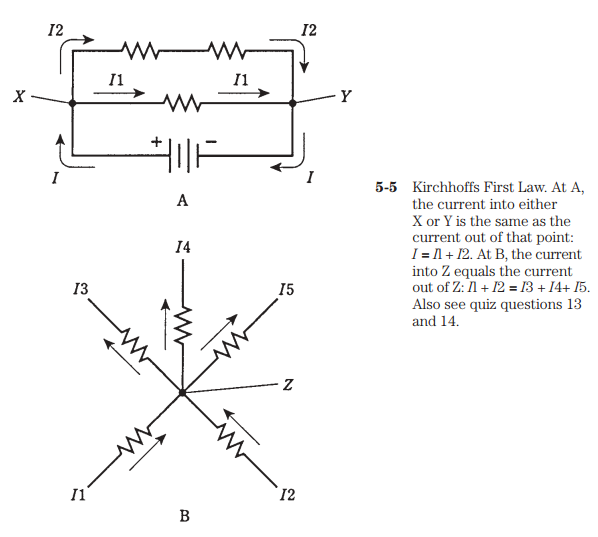13. Refer to Fig. 5-5A. Suppose the resistors each have values of 33 Ω. The battery provides 24 V. The current I1 is:

• A. 1.1 A.
• B. 730 mA.
• C. 360 mA.
• D. Not determinable from the information given.

14. Refer to Fig. 5-5B. Let each resistor have a value of 820 Ω. Suppose the top three resistors all lead to light bulbs of the exact same wattage. If I1 = 50 mA and I2 = 70 mA, what is the power dissipated in the resistor carrying current I4?

• A. 33 W.
• B. 40 mW.
• C. 1.3 W.
• D. It can’t be found using the information given.

NOTE: For questions 15 and 16 see Fig. 5-6.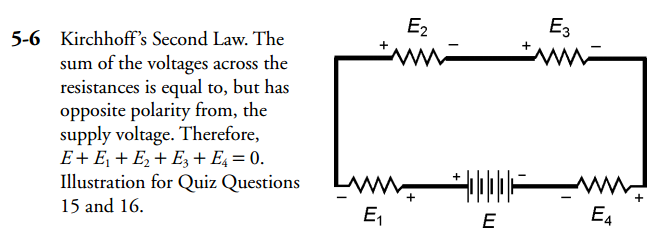15. Refer to Fig. 5-6. Suppose the resistances R1, R2, R3, and R4 are in the ratio 1:2:4:8 from left to right, and the battery supplies 30 V. Then the voltageE2 is:

• A. 4 V.
• B. 8 V.
• C. 16 V.
• D. Not determinable from the data given.

16. Refer to Fig. 5-6. Let the resistances each be 3.3 KΩand the battery 12 V. If the plus terminal of a dc voltmeter is placed between R1 and R2 (with voltages E1 and E2), and the minus terminal of the voltmeter is placed between R3 and R4 (with voltages E3 and E4), what will the meter register?

• A. 0 V.
• B. 3 V.
• C. 6 V.
• D. 12 V.

17. In a voltage divider network, the total resistance:

• A. Should be large to minimize current drain.
• B. Should be as small as the power supply will allow.
• C. Is not important.
• D. Should be such that the current is kept to 100 mA.

18. The maximum voltage output from a voltage divider:

• A. Is a fraction of the power supply voltage.
• B. Depends on the total resistance.
• C. Is equal to the supply voltage.
• D. Depends on the ratio of resistances.

NOTE: For questions 19 and 20 see Fig. 5-7.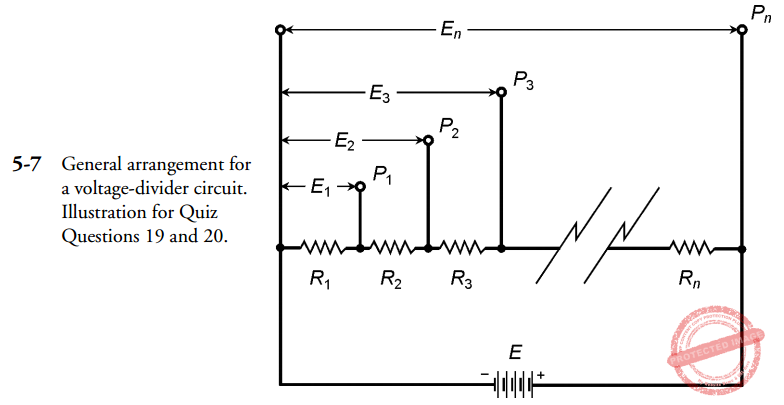19. Refer to Fig. 5-7. The battery E is 18.0 V. Suppose there are four resistors in the network: R1 = 100 Ω, R2 = 22.0 Ω, R3 = 33.0 Ω, R4 = 47.0 Ω. The voltage E3 at P3 is:

• A. 4.19 V.
• B. 13.8 V.
• C. 1.61 V.
• D. 2.94 V.

20. Refer to Fig. 5-7. The battery is 12 V; you want intermediate voltages of 3.0, 6.0 and 9.0 V. Suppose that a maximum of 200 mA is allowed through the network. What values should the resistors, R1, R2, R3, and R4 have, respectively?

• A. 15 Ω, 30 Ω, 45 Ω, 60 Ω.
• B. 60 Ω, 45 Ω, 30 Ω, 15 Ω.
• C. 15 Ω, 15 Ω, 15 Ω, 15 Ω.
• D. There isn’t enough information to design the circuit.

Rate this: